Next: B-splines Up: No Title Previous: Ray tracing primitives

Subsections

# Bezier curves

A Bezier curve is a weighted sum of n+1 control points,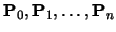, where the weights are the Bernstein polynomials: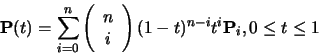(74)

The Bezier curve of order n+1 (degree n) has n+1 control points. These are the first three orders of Bezier curve definitions.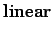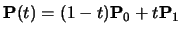(75)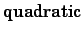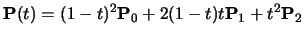(76)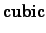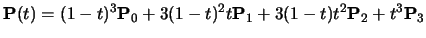(77)

## Ways of thinking about Bezier curves

There are several useful ways in which you can think about Bezier curves. Here are the ones that I use.
Linear interpolation.
Equation 75 is obviously a linear interpolation between two points. Equation 76 can be rewritten as a linear interpolation between linear interpolations between points: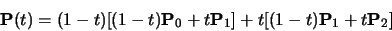(78)

Equation 77 can be rewritten as a linear interpolation between linear interpolations between linear interpolations between points. This is left as an exercise for the reader.
Weighted average.
A Bezier curve can be seen as a weighted average of all of its control points. Because all of the weights are positive, and because the weights sum to one, the Bezier curve is guaranteed to lie within the convex hull of its control points.
Refinement of the control polygon.
A Bezier curve can be seen as some sort of refinement of the polygon made by connecting its control points in order. The Bezier curve starts and ends at the two end points and its shape is determined by the relative positions of the n-1 other control points, although it will generally not pass through these other control points. The tangent vectors at the start and end of the curve pass through the end point and the immediately adjacent point.
Rogers and Adams list the properties of the Bezier curve on page 291.

## Continuity

You should note that each Bezier curve is independent of any other Bezier curve. If we wish two Bezier curves to join with any type of continuity, then we must explicitly position the control points of the second curve so that they bear the appropriate relationship with the control points in the first curve.

Any Bezier curve is infinitely differentiable within itself, and is therefore continuous to any degree (Cn-continuous,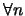). We therefore only need concern ourselves with continuity across the joins between curves. Assume that we have two Bezier curves of the same order: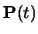, defined by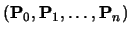, and, defined by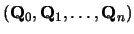. C0-continuity (continuity of position) can be achieved by setting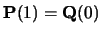. This gives a formula for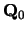in terms of the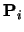s: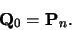(79)

Similarly for C1-continuity, we need C0-continuity and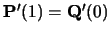, giving: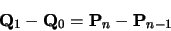(80)

Combining Equations 80 and 79 gives a formula for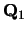in terms of thes:=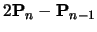(81) =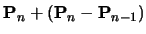(82)

Continuing in this vein, we find that the requirements for C2-continuity (i.e. C1-continuity and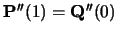) give: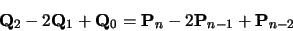(83)

Combining Equations 83, 80, and 79 gives a formula for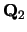in terms of thes:=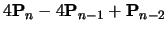(84) =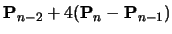(85)

## Bezier surfaces

We learnt in the IB course that the simplest way to construct a Bezier surface is as the tensor product of Bezier curves. A tensor product Bezier surface of order n+1 is defined by (n+1)2 control points. It is called a Bezier patch.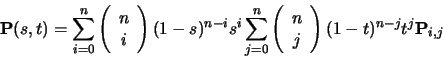(86)

You can think about this as moving the control points of one Bezier curve along a set of Bezier curves to sweep out a surface. Continuity across a boundary between two Bezier patches is only guaranteed if each of the Bezier curves across the join obey the curve continuity conditions. Again, this was covered in the IB course.Next: B-splines Up: No Title Previous: Ray tracing primitives
Neil Dodgson
2000-09-25